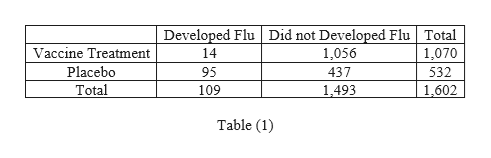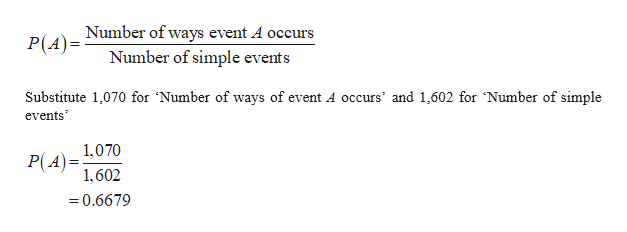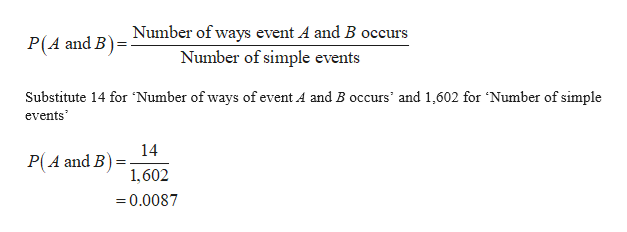# Did Not Develop FluDeveloped Flu1056Vaccine Treatment1495437Placebo

Question
2 views

In Exercises 6–10, use the following results from tests of an experiment to test the effectiveness of an experimental vaccine for children ( based on data from USA Today). Express all probabilities in decimal form

Find the probability of randomly selecting 1 of the subjects and getting 1 who developed flu, given that the subject was given the vaccine treatment.

check_circle

Step 1

The total number of childrenfor ‘an experiment to test the effectiveness of an experimental vaccine’ is obtained as shown below in Table (1).help_outlineImage TranscriptioncloseDeveloped Flu Did not Developed Flu 1,056 Total Vaccine Treatment 14 1,070 Placebo 95 437 532 Total 109 1,493 1,602 Table (1) fullscreen
Step 2

Let event A denote the probability of getting a child who had vaccine treatment and event B denote the probability of getting a child who had developed flu.

Probability:

The formula for probability of event A is,help_outlineImage TranscriptioncloseNumber of ways event A occurs Number of simple events P(A)= Substitute 1,070 for 'Number of ways of event A occurs' and 1,602 for 'Number of simple events' 1,070 P(A)= 1,602 = 0.6679 fullscreen
Step 3

The formula for probability of event ...help_outlineImage TranscriptioncloseNumber of ways event A and B occurs P(A and B) Number of simple events Substitute 14 for 'Number of ways of event A and B occurs' and 1,602 for Number of simple events 14 P(A and B) 1,602 = 0.0087 fullscreen

### Want to see the full answer?

See Solution

#### Want to see this answer and more?

Solutions are written by subject experts who are available 24/7. Questions are typically answered within 1 hour.*

See Solution
*Response times may vary by subject and question.
Tagged in

### Statistics